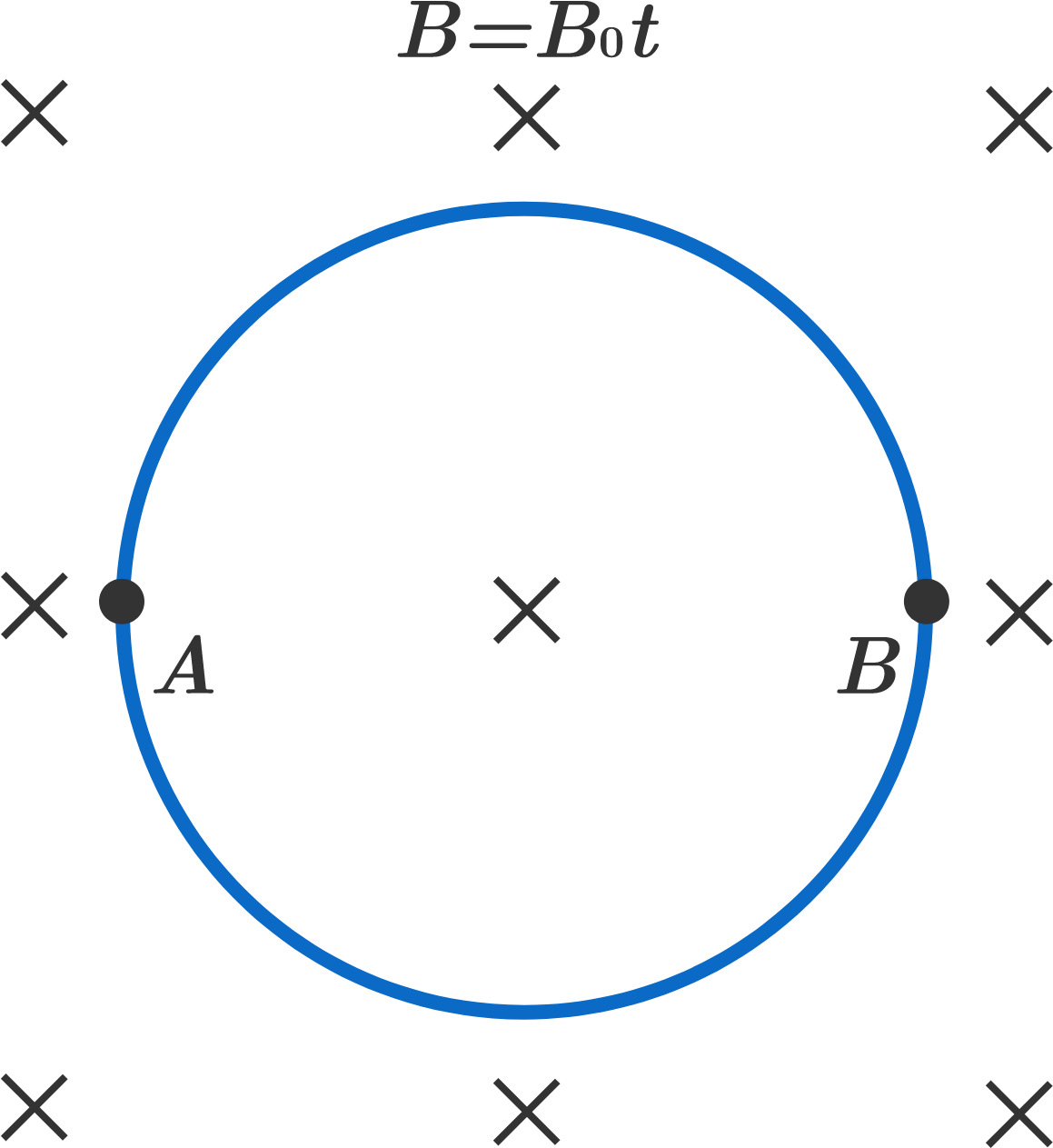# EMF vs Potential difference

A circular loop of radius $R$ is placed in a region of uniform time varying magnetic field $B=B_0t$. The magnetic field is into the plane of the ring. There are two points $A$ and $B$ diametrically opposite to each other on the ring, as shown in the diagram.What is the potential difference between points $A$ and $B$?


Details and Assumptions:

• The circular loop has uniform thickness and resistivity.
• $B_0$ is a constant and $t$ is time.
×